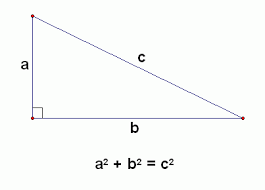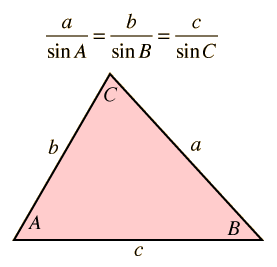## Trigonometry Class 10:

Trigonometry is a branch of mathematics dealing with relations involving lengths and angles of triangles. It can, in a simpler manner, be called the study of triangles. The angles are either measured in degrees or radians. We need to look into trigonometric formulae, ratios, functions etc. in order to grasp the concept completely. Here, we have discussed the basic concepts of Trigonometry Class 10.

## Triangle and their types

A triangle is a well-defined bounded figure consisting of three sides and three internal angles that add up to 180 degrees. The types of triangles can be divided on the basis of length and angle.
On the basis of length, there are three types: scalene (all the sides and angles are different), isosceles (2 sides and 2 angles are equal) and equilateral triangle (all the sides and angles are equal). On the basis of angles, it is again divided into three kinds: acute-angled triangle (all angles are less than 90 degrees), right-angled triangle (one angle is equal to 90 degrees) and obtuse-angled triangle (one angle is greater than 90 degree).

### Some basic points to remember for Trigonometry class 10

1. The sum of the three angles of the triangle equals 180 degree
2. The sum of the length of two sides of a triangle is greater than the third side whereas the difference between the lengths of two sides is less than the third side’s length.
3. The longest side is equal to the largest angle and vice versa.
4. Area of the triangle = ½ x base x height
5. According to Heron’s formula, Area of the triangle is √s(s−a)(s−b)(s−c), where s is the semi perimeter and is calculated by the following formula: ½ (a + b + c) where a, b, c, are the sides of the triangle.

Sine, Cosine and TangentWhen the angle shown is mentioned, the formulae for sine, cosine and tangent are:

Sine: Opposite / Hypotenuse

Tangent: Sine / Cosine = Opposite / Adjacent

Example, Calculate the angle in a right triangle with the measure of adjacent side being 12 cm and hypotenuse being 20 cm:

The adjacent side = 12 cm

Hypotenuse = 20 cm

= 12 / 20

cos θ  =  12 / 20

θ = cos−1 (0.6)

θ = 99.99

In such a case, sometimes we might need to calculate Cosec, Sec and cot too. In such cases, the formulae to be used are:
Cosec (x) = 1 / sine (x)

Sec (x) = 1 / cos (x)

Cot (x) = 1 / tan (x)

## Pythagoras theorem and some other identities

• This is an extremely important theorem for trigonometry class 10. The theorem states that the sum of squares of the base and height of a right triangle is equal to the square of the hypotenuse.According to the theorem

• a2 + b2 = c2
• Sin ² θ + Cos ² θ = 1
• Tan 2 θ + 1 = Sec2 θ
• Cot2 θ + 1 = Cosec2 θ
• Sin 2θ = 2 sin θ cos θ
• Cos 2θ = cos² θ – sin² θ
• Tan 2θ = 2 tan θ / (1 – tan² θ)
• Cot 2θ = (cot² θ – 1) / 2 cot θ

## Congruence of a triangle

If a triangle is such, that when it is placed on the other, can be superimposed on it, it is said to be a set of congruent triangles. If the sides and angles of two triangles are the same, then they are called congruent triangles. It is denoted by the sign ≅

There are different rules for congruency. They are:

1. SSS (Side Side Side) – If the three sides of triangle 1 are equal to the corresponding sides of triangle 2, they are said to be congruent by the SSS property.
2. SAS (Side Angle Side) – If any two sides of a triangle 1 and their included angle are equal to the corresponding two sides and the respective included angle of triangle 2, they are said to be congruent by the SAS property.
3. ASA (Angle Side Angle) – If any two angles and the included side of triangle 1 are equal to the corresponding two angles and the included side of triangle 2, they are said to be congruent by the ASA property.
4. RHS (Right-Angle Hypotenuse Side) – If a side and hypotenuse of a right triangle 1 is equal to the corresponding side and hypotenuse of a right triangle 2, then they are said to be congruent by the RHS property.

## Similar Triangles

Triangles with same angles and different length of sides are called similar triangles. They are denoted by ~ symbol. Hence, for two triangles to be similar the conditions that need to be met are:

• Corresponding angles are equal
• Corresponding sides are proportionateThese two triangles are similar if and only if:

• ∠A = ∠X, ∠B = ∠Y and ∠C = ∠Z
• AB / XY = BC / YZ = AC / XZ

There are different rules for the similarity of a triangle. They are:

• AAA

If any two angles of triangle 1 are equal to corresponding angles of triangle 2, they are similar triangles by the AAA property.

• SAS

If two sides of triangle 1 is in the same ratio of the two angles of triangle 2 and the angle included by the 2 sides are equal in both the triangle, it is said to be similar by the SAS property.

• SSS

If the three sides of triangle 1 are in proportion to the three sides of triangle 2 they are said to be similar by SSS property.

### Some theorems on similarity

The ratio of area of two triangles is in proportion with the squares of ratio of their sides, if two triangles are similar. In triangle ABC and triangle PQR, if they are similar, then

Area of ABC / Area of PQR = (AB/PQ)2 = (BC/QR)2 = (CA/RP)2

### Law of SineThe law states that:
a / sin A = b / sin B = c / sin C

Here were the basic that you need to know about Trigonometry Class 10. To know about Trigonometric Formulas, visit here!

+91
No thanks.

## Request a Free 60 minute counselling session at your home

Please enter a valid phone number
•7,829,648

Happy Students
•358,177,393

Questions Attempted
•3,028,498

Tests Taken
•3,020,367